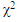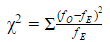PURCHASE A DIGITAL COPY PURCHASE A HARD COPY Lesson 1 Introduction to Statistical Research Methods Lesson 2 Visualizing Data Lesson 3 Central Tendency Lesson 4 Variability Lesson 5 Standardizing Lesson 6 Normal Distribution Lesson 7 Sampling Distributions Lesson 8 Estimation Lesson 9 Hypothesis Testing Lesson 10 t-Tests for Dependent Samples Lesson 11 t-Tests for Independent Samples Lesson 12 Intro to One-Way ANOVA Lesson 13 One-Way ANOVA: Test significance of differences Lesson 14 Correlation Lesson 15 Linear Regression Lesson 16 Chi-Squared Tests Afterward Index

All the tests we’ve done so far have involved sets of data for which we could find the mean and standard deviation. However, sometimes we only have frequencies or proportions. For example, let’s say that marketing researchers post an article about a new product on Facebook, Twitter, LinkedIn, and Instagram. They want to determine if followers are more likely to read the article from a particular social network.

After releasing the article, the marketing researchers found the following numbers of people clicked the link:z-tests and t-tests are parametric tests since they’re based on means and standard deviations. In this case, we need to do a non-parametric test to
determine if the number of people who clicked the link to the article is what we would have expected based on the number of followers on each social
network. This is the null hypothesis; the alternative hypothesis is that the number of people who clicked the link is different than what was expected.

In this case, we’ll do a chi-squared goodness-of-fit testto test the “fit” between observed and expected values. This involves computing astatistic:To continue learning, purchase Street-Smart Stats: A Friendly Introduction to Statistical Research Methods.